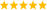# LabVIEW

cancel
Showing results for
Did you mean:

Solved!
Go to solution

## A question about multi conditions if statement

Hello everyone!

I'm sorry if this is a simple question. I'm actually new to LabVIEW.

I wonder what is the best way to solve this problem in my code:

I have 3 1D array each contains simple numbers.

I have written a case structure which I intend to be executed only if there a predefined max values in all 3 arrays.

in simple word:

if max in array1 > A && max in array2 > B && max in array3 > C >> execute case structure.

How should I do it?

Thanks.

Fernando.

Message 1 of 6
(272 Views)

## Re: A question about multi conditions if statement

@Fernando68 wrote:

Hello everyone!

I'm sorry if this is a simple question. I'm actually new to LabVIEW.

I wonder what is the best way to solve this problem in my code:

I have 3 1D array each contains simple numbers.

I have written a case structure which I intend to be executed only if there a predefined max values in all 3 arrays.

in simple word:

if max in array1 > A && max in array2 > B && max in array3 > C >> execute case structure.

How should I do it?

Thanks.

Fernando.

Well,  you seem to have driven the condition all the way down to TWO CASES.. .... Select is an option.   Wiring that boolean output to a Case Structure's selection node is another option.

Message 2 of 6
(258 Views)
Solution
Accepted by topic author Fernando68

## Re: A question about multi conditions if statement

Maybe something like that?(built array, FOR loop, array max, greater?, AND array elements, case structure)

The above assumes that the three arrays are of equal size. If the sizes can be different, a little bit more code is needed, especially if the max is <0.LabVIEW Champion. It all comes together inGCentralMessage 3 of 6
(246 Views)

## Re: A question about multi conditions if statement

Thank you!

I don't know if I should make another thread for this question or not.

Let's say each array contains one column with 500 rows of numbers and the max value is non-zero.

And let's suppose that the max value of the Array1 is in index number 300 (300,1).

How can I execute the case structure only if the Array2, and Array3  max values are in a predefined indexes before of after the index value of the Array1 (For example from index number 250 to 350)?

I want to define a window where if the second and third arrays have a predefined max values above an specific number in that window compared the first array ( which can be any of the 3 arrays ) then the case structure should be executed.

Thanks again.

Fernando.

Message 4 of 6
(141 Views)

## Re: A question about multi conditions if statement

Thank you!

I think I need to make another thread for this question but let me ask it anyway.

What if I go one step further.

Let's suppose that each array contains one column with 500 rows of simple numbers and they have same sizes.

And let's suppose that the max value of each array is non-zero but different from another one.

Let's suppose that the max value of (for example) Array2 is above B and is in the index number 300 (300,1) , How can I set the case structure to be executed only if the max value of Array1>A and max Array3>C "AND" index numbers of max of Array1 and max of Array3 are from 250-350 ?

I what to define a windows where the case structure should be executed only if the max value of all three arrays are above predefined value "and" inside that window compared the the index number of one particular array.

Thanks.

Fernando

Message 5 of 6
(168 Views)

## Re: A question about multi conditions if statement

When you get the index of the max, put it through the in range and coerce function to see if it is IN RANGE of your desired values.  Now you have a boolean.

Use that with the boolean logic you are already using.

Message 6 of 6
(145 Views)